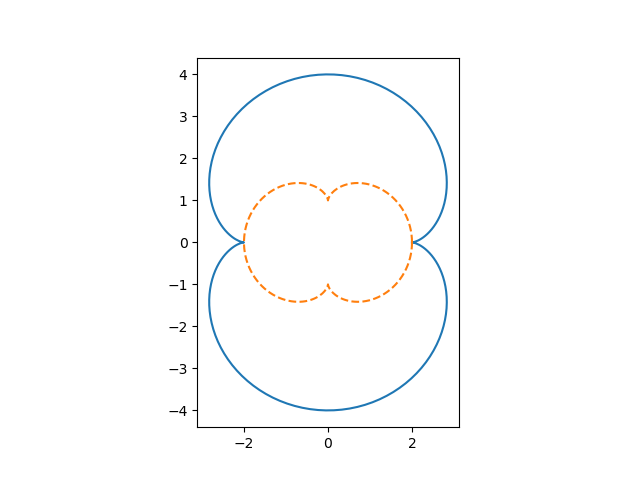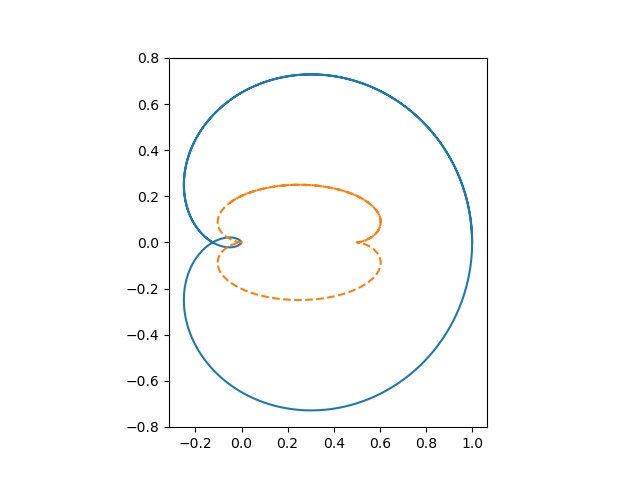# Nephroids and evolutes

The previous post looked at the evolute of an ellipse. This post will look at evolutes more generally, and then look at nephroids.

As a quick reminder, given a curve c, a point on its evolute is the center of curvature for a point on c. See the previous post for a detailed example.

If a curve has a parameterization (x(t), y(t))T then its evolute has parameterization## Nephroid curve

Let’s apply this to a nephroid. This word comes from the Greek for kidney-shaped. Comes from the same root as nephrology.

A nephroid can be parameterized byWhen we compute the evolute for this curve we getIt’s not apparent from the expression above that the evolute of an nephroid is another nephroid, but it is clear from the plot below.The solid blue curve is the nephroid, and the dashed orange curve is its evolute. Apparently the evolute is another nephroid, rotated and rescaled. We’ll come back to the equations shortly, but let’s look at another example first.

## Cayley’s sextit

There is a curve known as Cayley’s sextit that has parameterizationWhen we compute the evolute of this curve we getWhen we plot Cayley’s sextit and its evolute we getNote that to get the full curve of the evolute we let t run from 0 to 4π.

Again it’s not at all obvious from the equations, but apparently the evolute of Cayley’s sextit is also a nephroid, this time scaled, stretched, and shifted.

This says that pre-evolutes are not unique: two different curves can have the same (family) of evolutes.

## Algebra

Now for the hard part: doing the algebra to show that the curves that look like nephroids are indeed nephroids.

We need to show that the set of points traced out by the evolute is a set of points on a nephroid: we don’t have to show that our parameterization of the evolute can be turned into our parameterization of a nephroid. So we shift to an implicit equation for a nephroid, scaled by a factor a:### Nephroid evolute

First let’s show that the evolute of a nephroid is a nephroid. From the plot it appears that the evolute is scaled by 1/2 and rotated a quarter turn. So we suspect that if we reverse x and y and multiply both by 1/2 we’ll get a nephroid. Let’s let Mathematica verify this for us:

    implicit[x_, y_, a_] := (x^2 + y^2 - 4 a^2)^3 - 108 a^4 y^2
Simplify[implicit[(2 + Cos[2 t]) Sin[t], 2 Cos[t]^3, 1/2]]


Without calling Simplify we get something that isn’t obviously zero, but applying trig identities can reduce it to zero.

### Cayley’s sextit evolute

In order to show that the evolute of Cayley’s sextit is a nephroid, we have to find what nephroid we believe it is.

Let’s look at the our parameterization of a nephroid again:We can see that the two cusps are at (-2, 0) and (2, 0), and the minimum and maximum heights are (0, -4) and (0, 4). We’ll line these points up with their counterparts in the evolute to Cayley’s sextit. Let’s look at its parameterization.The two cusps correspond to y = 0, which happens when t = 0 and t = 3π/2. The corresponding x values are 1/2 and 0. This suggests the center of our nephroid is at 1/4 and our nephroid has been scaled by 1/8 in the horizontal direction.

The top and bottom correspond to x = 1/4, which happens when t = 3π/4 and t = 9π/4, and so our nephroid has height 1/4. This says our nephroid has been scaled by 1/16 in the vertical direction.

So if we shift x by 1/4 then multiply by 8, and multiply y by 16, we suspect we’ll get a standard nephroid. Let’s see what Mathematica has to say.

    implicit[8(-Cos[t/3]^2 (-2 Cos[2 t/3] + Cos[4 t/3])/2 - 1/4),
16 Sin[2 t/3]^3/4, 1]


This confirms that our guesses were correct. The evolute of Cayley’s sextit is indeed a nephroid, and we’ve identified which nephroid it is.